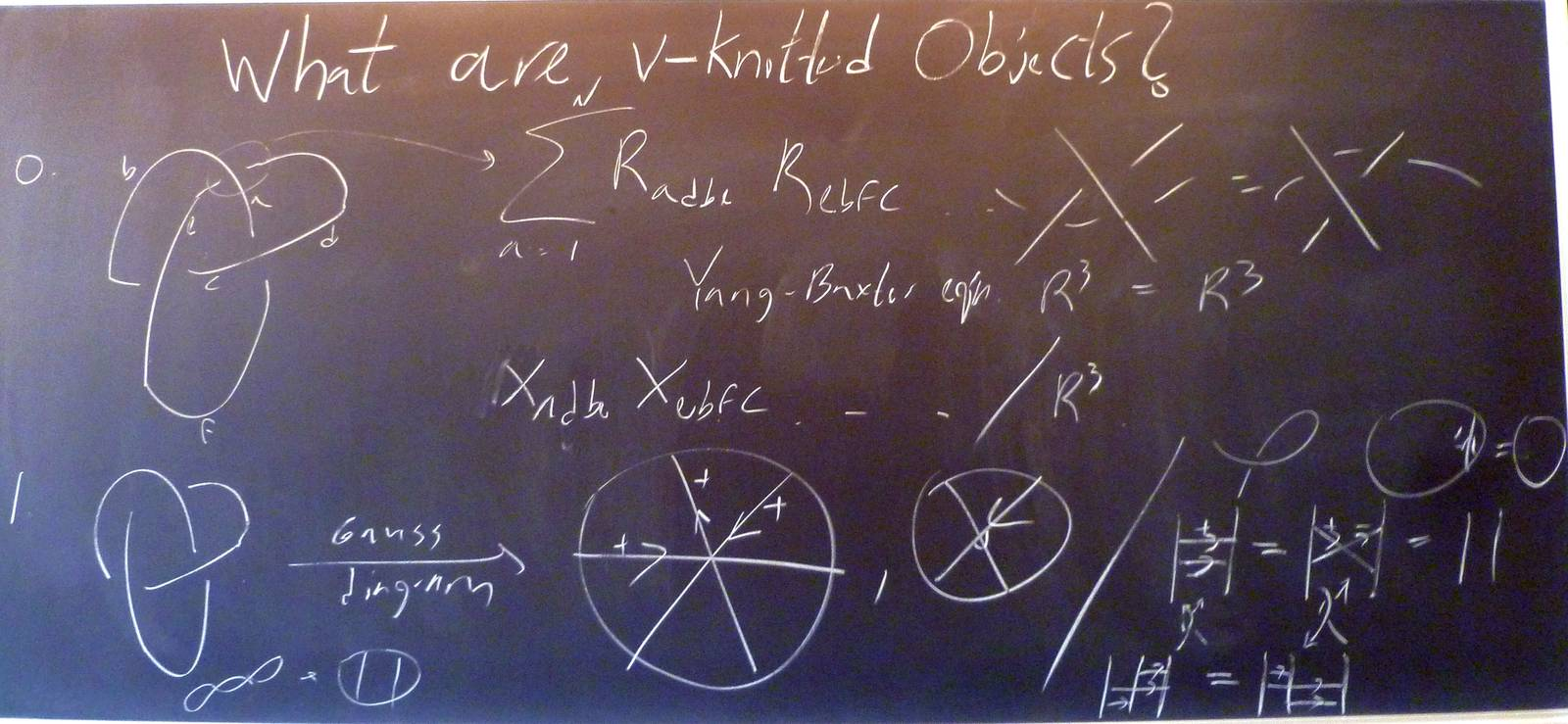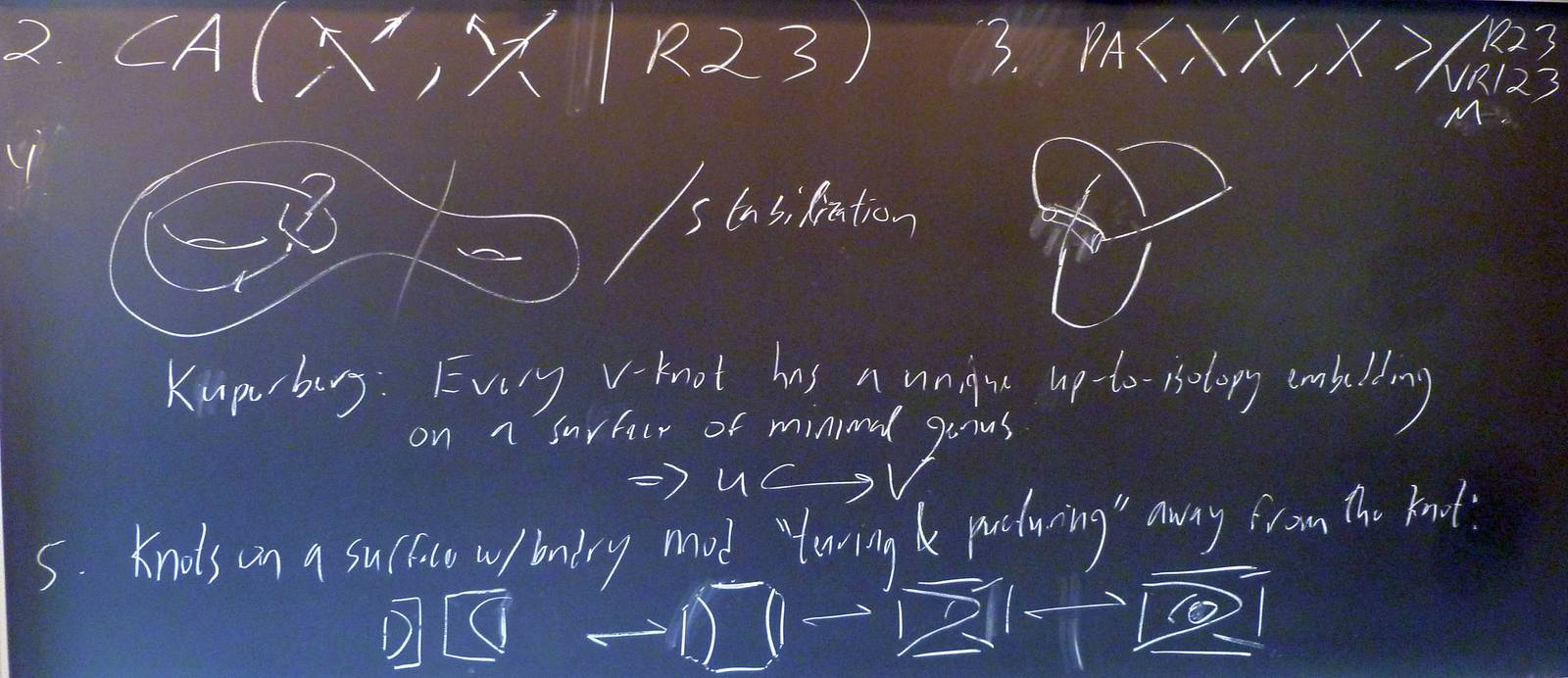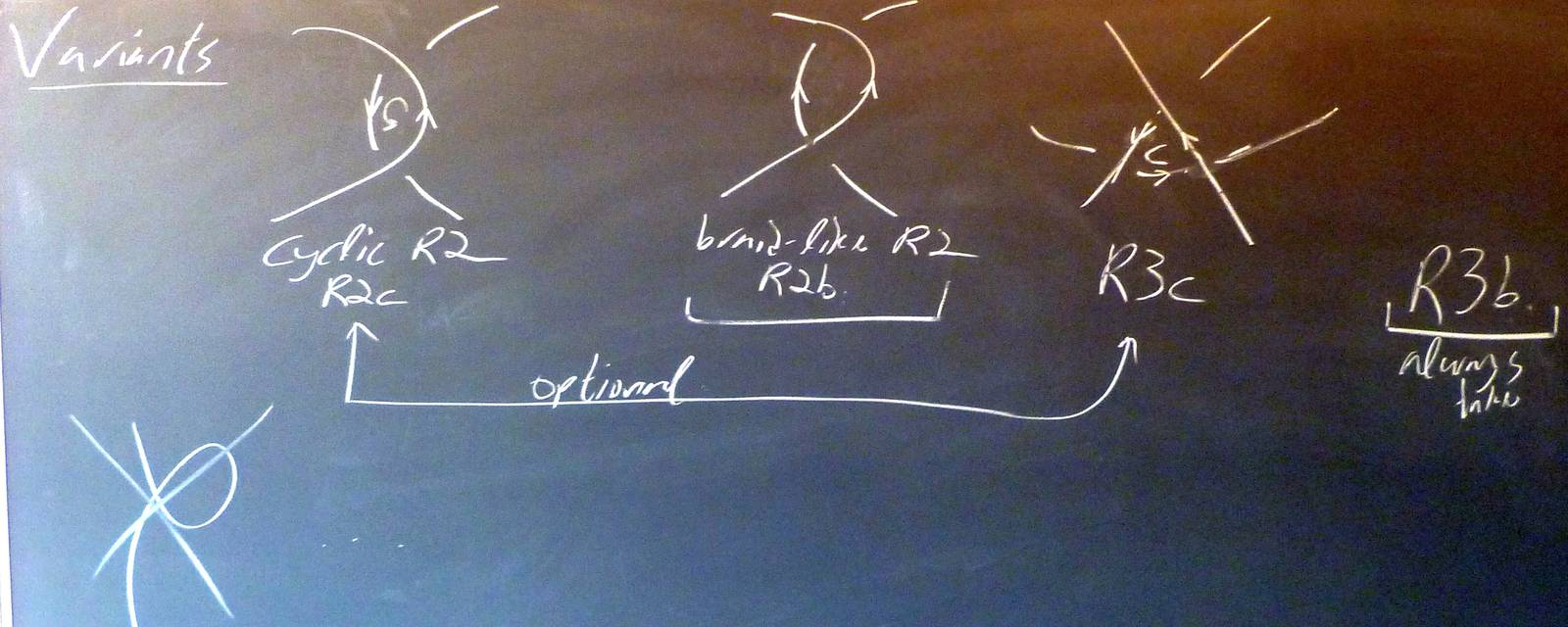© | Dror Bar-Natan: Talks/Classes: Aarhus-1305 < >

# Day 9 Clip 1

 width: 400 832 download ogg/Aarhus-130607-1_400.ogg For now, this video can only be viewed with web browsers that support

Notes on Aarhus-130607-1:    [edit, refresh]

refresh
panel
Managed by dbnvp: The small-font numbers on the top left of the videos indicate the available native resolutions. Click to test.

0:16:23 [add] What are v-knotted objects?0:17:09  But I didn't say it precisely enough - I said it wrong. The correct statement is that if two knots $\gamma_1$ and $\gamma_2$ embedded on surfaces $\Sigma_1$ and $\Sigma_2$ are equivalent as virtual knots, and if $\Sigma_1$ and $\Sigma_2$ are both of genus $g$ where $g$ is the minimal genus on which $\gamma_{1,2}$ may be embedded, then there is a diffeomorphism $f\colon\Sigma_1\to\Sigma_2$ such that $f(\gamma_1)$ is isotopic to $\gamma_2$.
0:29:10 [add] What are v-knotted objects? (2)0:40:57 [add] Variants for v-knotted objects.